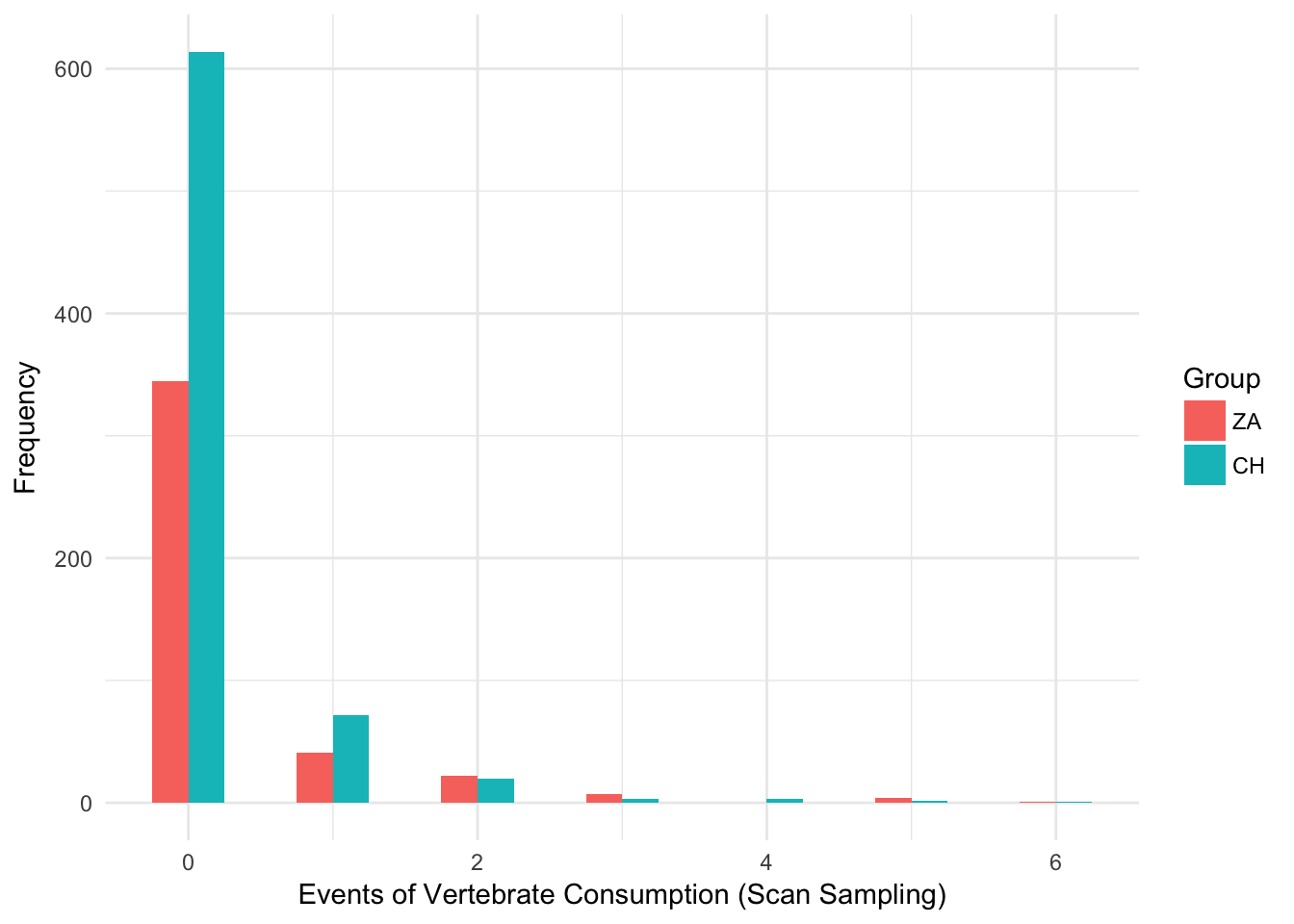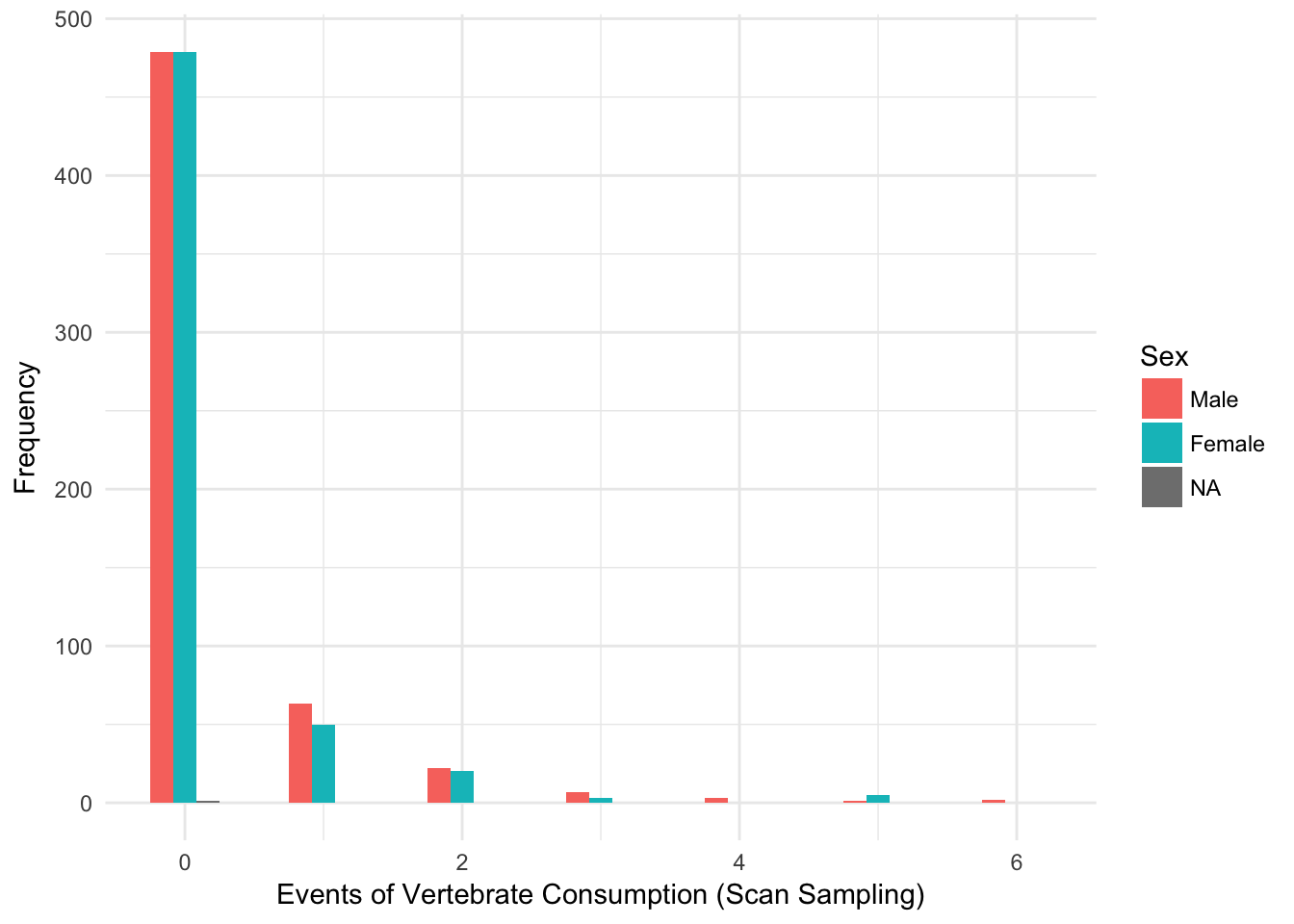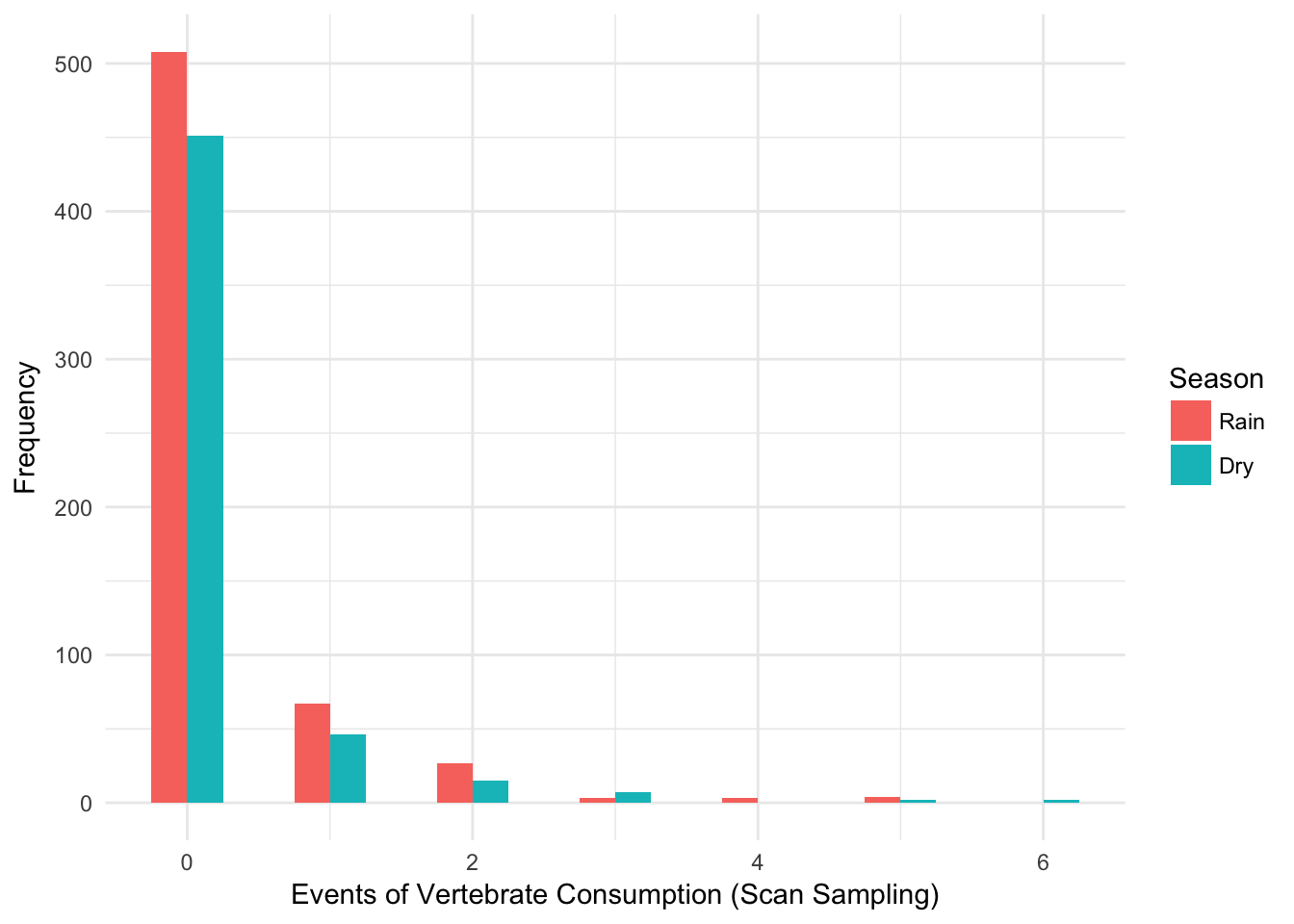## Vertebrate consumption by wild bearded capuchin monkeys (Sapajus libidinosus) living in a Brazilian semiarid habitat

#### Short report from a data analysis in R

Let’s pull all data

SSdata <- read.csv2("/Users/del/ACADEMY/WIP/CVP/data2SS.csv") # Reading dataset with Scan Sampling raw data
# test <- read.spss("/Users/del/ACADEMY/WIP/CVP/vert.sav", to.data.frame=TRUE) # Reading SPSS file, it has many errors (e.g. misses a line, Month is not a date, etc.)

## Data cleaning

Some data cleaning in order to improve readability

SSdata$Group <- as.factor(SSdata$Group)
AOdata$Group <- as.factor(AOdata$Group)
levels(SSdata$Group) <- c('ZA', 'CH') levels(AOdata$Group) <- c('ZA', 'CH')
SSdata$Sex <- as.factor(SSdata$Sex)
levels(SSdata$Sex) <- c('Male', 'Female') SSdata$Age <- as.factor(SSdata$Age) levels(SSdata$Age) <- c('Adult', 'Juvenile', 'Infant')
SSdata$Season <- as.factor(SSdata$Season)
levels(SSdata$Season) <- c('Rain', 'Dry') SSdata_without_infants <- SSdata[SSdata$Age != 'Infant',] # separate dataset without infants
SSdata_without_infants$Age <- droplevels(SSdata_without_infants$Age)

## Exercises

Correct and fill: A total of 474 events of vertebrate consumption were observed between 2006 and 2010 (274 recorded by scan sampling and 200 recorded by all occurrences), CH group accounted for XX events, and ZA group for XX events.

sum(SSdata$Scan.consumed.month) + sum(AOdata$TotalEpisodes)
##  446
sum(SSdata$Scan.consumed.month) ##  281 sum(AOdata$TotalEpisodes)
##  165
sum(AOdata$TotalEpisodes[AOdata$Group == 'CH']) + sum(SSdata$Scan.consumed.month[SSdata$Group == 'CH'])
##  212
sum(AOdata$TotalEpisodes[AOdata$Group == 'ZA']) + sum(SSdata$Scan.consumed.month[SSdata$Group == 'ZA'])
##  234

A total of 446 events of vertebrate consumption were observed between 2006 and 2010 (281 recorded by scan sampling and 165 recorded by all occurrences), CH group accounted for 212 events, and ZA group for 234 events.

Correct and fill: Of 213 episodes recorded by all occurrences for both groups, 81.2% (N=XX) were episodes in which only one individual was seen consuming the prey, whereas XX% (N=XX) were episodes in which two or more monkeys were seen consuming the same prey;

sum(AOdata$IndEpisodes)/sum(AOdata$TotalEpisodes)*100 # percent
##  81.21212
sum(AOdata$IndEpisodes) # n ##  134 sum(AOdata$MultipleIndEpisodes)/sum(AOdata$TotalEpisodes)*100 # percent ##  18.78788 sum(AOdata$MultipleIndEpisodes) # n
##  31

Of 165 episodes recorded by all occurrences for both groups, 81.21% (N=134) were episodes in which only one individual was seen consuming the prey, whereas 18.79% (N=31) were episodes in which two or more monkeys were seen consuming the same prey;

Correct and fill: The major prey types were reptiles (n=131: lizards, geckos, snakes, iguanas), mammals (n=95: primarily rodents, but also opossums, a bat) and birds (n=34: especially nestlings and eggs).

sum(c(SSdata$Lizards, SSdata$Serpents)) # reptiles
##  136
sum(c(SSdata$Rodents, SSdata$MarsupialsMammals, SSdata$Chiroptera)) # mammals ##  97 sum(SSdata$Aves) # birds
##  34

The major prey types were reptiles (n=136: lizards, geckos, snakes, iguanas), mammals (n=97: primarily rodents, but also opossums, a bat) and birds (n=34: especially nestlings and eggs).

Calculate percentages for Table 2

Table 2 in the Word file has many errors. Here I recalculate all values for paper-corrections.

str(SSdata[,9:15])
## 'data.frame':    1135 obs. of  7 variables:
##  $Lizards : int 0 0 0 1 4 0 0 0 0 2 ... ##$ Serpents         : int  0 0 0 0 0 0 0 0 0 0 ...
##  $Rodents : int 0 0 0 0 0 0 0 0 0 0 ... ##$ MarsupialsMammals: int  0 0 0 0 0 0 0 0 0 0 ...
##  $Chiroptera : int 0 0 0 0 0 0 0 0 0 0 ... ##$ Aves             : int  0 0 0 0 0 0 0 0 0 0 ...
##  $Undertermined : int 0 0 0 0 1 0 0 0 0 0 ... sum(SSdata$Lizards)/sum(SSdata[,9:15])*100
##  43.77224
sum(SSdata$Serpents)/sum(SSdata[,9:15])*100 ##  4.626335 sum(SSdata$Rodents)/sum(SSdata[,9:15])*100
##  28.46975
sum(SSdata$MarsupialsMammals)/sum(SSdata[,9:15])*100 ##  5.338078 sum(SSdata$Chiroptera)/sum(SSdata[,9:15])*100
##  0.7117438
sum(SSdata$Aves)/sum(SSdata[,9:15])*100 ##  12.09964 sum(SSdata$Undertermined)/sum(SSdata[,9:15])*100
##  4.982206
sum(SSdata[,9:15])
##  281

## Visualization

#### Differences of vertebrate consumption (from SS data) amongst groups

ggplot(SSdata, aes(Scan.consumed.month, fill = Group)) +
geom_histogram(binwidth=.5, position="dodge") +
labs(x = 'Events of Vertebrate Consumption (Scan Sampling)', y = 'Frequency') +
theme_minimal()#### Differences of vertebrate consumption (from SS data) amongst Sex

ggplot(SSdata, aes(Scan.consumed.month, fill = Sex)) +
geom_histogram(binwidth=.5, position="dodge") +
labs(x = 'Events of Vertebrate Consumption (Scan Sampling)', y = 'Frequency') +
theme_minimal()#### Differences of vertebrate consumption (from SS data) amongst Seasons

ggplot(SSdata, aes(Scan.consumed.month, fill = Season)) +
geom_histogram(binwidth=.5, position="dodge") +
labs(x = 'Events of Vertebrate Consumption (Scan Sampling)', y = 'Frequency') +
theme_minimal()#### Differences of vertebrate consumption (from SS data) amongst Age groups

ggplot(SSdata, aes(Scan.consumed.month, fill = Age)) +
geom_histogram(binwidth=.5, position="dodge") +
labs(x = 'Events of Vertebrate Consumption (Scan Sampling)', y = 'Frequency') +
theme_minimal()